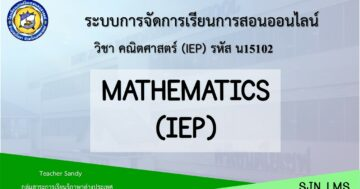# Mathematics IEP P.5 2nd Semester## เกี่ยวกับคอร์ส

Intensive Mathematics 5                                                                   Department of Mathematics
Primary 5                                                                                              Time 20 Hours

Students are able to write fractions which the denominators are composed of 10, 100 or
1,000 in the form of decimals; show method of finding answers by using the unitary method (rule
of three), find the sum, difference, product, and quotient of fractions and mixed numbers; show
method of finding answers to 2 step addition, subtraction, multiplication and division problems of fractions; multiply decimals with answers in decimals of not more than 3 places; divide the
dividends which are cardinal numbers or decimals of not more than 3 places and divisors are
cardinal numbers with answers in decimals of not more than 3 places; show method of finding
answers to 2-step addition, subtraction, multiplication and division word problems of decimals;
show method of finding answers to problems not more than 2 steps of percentages; show the
method of finding the answers to the problems involving the length and weight which unit is
changed and written in decimals; show the method of finding the answers to the problems
involving the volume of cuboids and the capacity of cuboids containers, perimeter of a
quadrilateral and the area of a parallelogram and rhombus; construct a line or a line segment to
be parallel with the given line or line segment; classify a quadrilateral by considering from its
properties; construct any quadrilateral when giving the length of each side and the size of the
angle or when giving the diagonal; tell the characteristics of a prism; use the information from a
line graph to find the answer for the problems; and write a bar chart from the information which
is cardinal number.

These can be done through the determined situations for the students to learn, observe,
exercise their skills through the practices, summaries; to have good attitude towards mathematics; to be creative; to develop their skills and mathematical interpretation; and be able to use in their daily life.

Indicators
M1.1      Gr5/1      Gr5/2      Gr5/3      Gr5/4      Gr5/5

Gr5/6      Gr5/7      Gr5/8      Gr5/9

M2.1      Gr5/1      Gr5/2      Gr5/3     Gr5/4

M2.2     Gr5/1      Gr5/2      Gr5/3      Gr5/4

M3.1      Gr5/1      Gr5/2

Total 19 indicators

### What Will You Learn?

• Students are able to think about numbers and can solve word problems.
• Students are able to develop their Mathematics skill.- Master of Education, Curriculum and Instruction, Phayao University, Thailand (2021) - Bachelor of Arts, English Major, Mae Fah Luang University, Thailand (2015) - Teacher of Intensive English Program (IEP) for 7 years - [Contact: Line -- sineyy]

00:00
00:00

00:00
00:00
00:00
00:00
00:00

00:00
00:00

00:00
00:00

00:00
00:00

### Student Ratings & ReviewsNo Review Yet